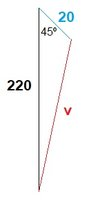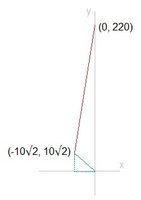# I need a little help

#### Benabob

##### New member
I can't quite figure this one out any help is appreciated

#### Attachments

• 4.1 MB Views: 24

#### firemath

##### Full Member
This is an adding vectors problem.

One vector travels straight down, (220cis270).
One travels northwest, (20cis135).

See if you can add these together.

#### MarkFL

##### Super Moderator
Staff member
This is an adding vectors problem.

One vector travels straight down, (220cis270).
One travels northwest, (20cis135).

See if you can add these together.
Rather than invoking the $$\cis(\theta)$$ function for complex numbers, I'd recommend standard vector notation instead. While the two are essentially mathematically equivalent, it may be confusing to students.

•Subhotosh Khan and firemath

#### Cubist

##### Junior Member
Be careful that winds are named by the direction they blow from.

You could start by drawing a sketch of the situation. Mark the departure point, and the arrival point. Then draw a vector of how far a balloon would be blown by the wind during 1h if it was released from the departure point. Can you then complete the problem?

Please assume the balloon travels at full wind speed and does not get caught in a tree•firemath

#### Otis

##### Senior Member
Hi Benebob. Can you show us what you've tried? I'd like to know what your drawing looks like. My first one has two triangles on the xy-plane, with the plane departing from the point (0,220). Knowing that a NW wind forms a right triangle with equal legs allowed me to determine the coordinates of where the plane would be one hour later if there were no wind. I then used the distance formula.

After reading post #4, I sketched the diagram suggested, and I got the same result using the law of cosines.

As suggested by others, one could also use vector arithmetic. What approach did you think about?#### firemath

##### Full Member
Sorry I left OP hanging. I got off the site early.

#### Otis

##### Senior Member
Just in case you want to check your diagram …
Hi hoosie. My diagram looks different. What answer did you get, for the exercise?#### Otis

##### Senior Member
To follow up, the diagram in post #6 doesn't show the plane arriving due south of its departure point (as stated in the exercise). Instead, it shows the plane arriving 220 miles due south of the point where the balloon (from post #4) would be at the plane's arrival time.

I drew the diagram described in post #4 like this and used the law of cosines.My first diagram looked like this, and I used the distance formula.(I used the Pythagorean Theorem, to determine the legs of the blue triangle.)

Expressing horizontal and vertical components of vectors, using those in some vector arithmetic and then calculating the magnitude of a resultant vector is probably the intent of the OP's assignment (they posted on the calculus board). I'll leave that demonstration for somebody else.Last edited:

#### hoosie

##### Junior Member
To follow up, the diagram in post #6 doesn't show the plane arriving due south of its departure point (as stated in the exercise). Instead, it shows the plane arriving 220 miles due south of the point where the balloon (from post #4) would be at the plane's arrival time.

I drew the diagram described in post #4 like this and used the law of cosines.

View attachment 16179

My first diagram looked like this, and I used the distance formula.

View attachment 16180

(I used the Pythagorean Theorem, to determine the legs of the blue triangle.)

Expressing horizontal and vertical components of vectors, using those in some vector arithmetic and then calculating the magnitude of a resultant vector is probably the intent of the OP's assignment (they posted on the calculus board). I'll leave that demonstration for somebody else.I stand corrected Otis - your diagram is indeed correct.